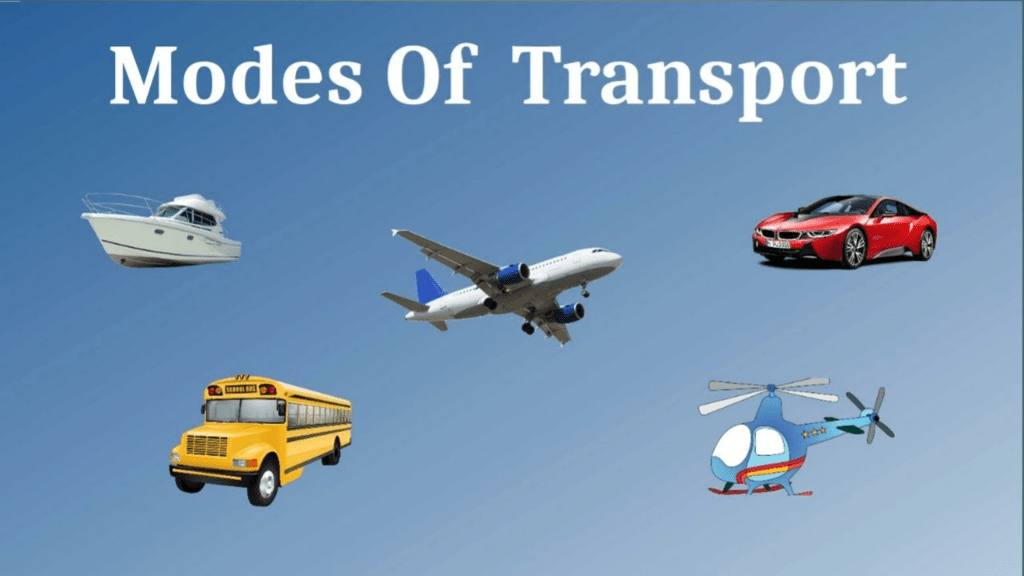UPSC  >  NCERT Solutions: Motion & Measurement of Distances

# Motion & Measurement of Distances NCERT Solutions - Science & Technology for UPSC CSE

Q1. Give two examples each, of modes of transport used on land, water and air.
Ans:
Land transport: Bus, train, motorbike.
Water transport: Boat, Ship, steamer.
Air Transport: Aeroplane, Helicopter.Q2. Fill in the blanks:
(i) One metre is ______________ cm.
(ii) Five kilometres is ______________ m.
(iii) Motion of a child on a swing is ______________.
(iv) Motion of the needle of a sewing machine is ______________.
(v) Motion of wheel of a bicycle is______________.
Ans:
(i) One metre is 100 cm.
(ii) Five kilometres is 5000 m.
(iii) The motion of a child on a swing is periodic motion.
(iv) The motion of the needle of a sewing machine is periodic motion.
(v) The motion of the wheel of a bicycle is circular motion.

Q3. Why can a pace or a footstep not be used as a standard unit of length?
Ans: A pace or a footstep not be used as a standard unit of length because the size of a pace and footstep vary from person to person. This will lead to confusion while measuring the lengths by different persons. We should use standard units like the International System of Units (SI Units).

Q4. Arrange the following lengths in their increasing magnitude:
1 metre, 1 centimetre, 1 kilometre, 1 millimetre.
Ans: 1 millimetre, 1 centimetre, 1 metre, 1 kilometre

Q5. The height of a person is 1.65 m. Express it into cm and mm.
Ans:  We know that,
1 metre = 100 cm
1 metre = 1000 mm
So, 1.65 m = 1.65 × 100
= 165 cm
1.65 m = 1.65 × 1000
= 1650 mm

Q6. The distance between Radha's home and her school is 3250 m. Express this distance into km.
Ans: We know that 1 m = 1/1000 km
So, 3250 m = 3250/1000 km
= 3.25 km

Q7. While measuring the length of a knitting needle, the reading of the scale at one end is 3.0 cm and at the other end is 33.1 cm. What is the length of the needle?
Ans: The length of the needle = 33.1 cm – 3.0 cm = 30.1 cm

Q8. Write the similarities and differences between the motion of a bicycle and a ceiling fan that has been switched on.
Ans:
Similarity: The blades of a fan and the wheels of a bicycle show circular motion.
Difference: Bicycles move in rectilinear motion, but the fan does not move in rectilinear motion.

Q9. Why would you not like to use a measuring tape made of an elastic material like rubber to measure distance? What would be some of the problems you would meet in telling someone about a distance you measured with such a tape?
Ans: An elastic measuring tape will not give accurate measurements as it stretches in length and reduces in size when stretched. When we express measurements taken with elastic tape, we have to tell whether the tape was stretched. If yes, how much? Hence, it is very difficult to tell the measurement taken from an elastic tape.

Q10. Give two examples of periodic motion.
Ans:

• Rotation of Earth
• Motion of Pendulum
• Vibrating wire of the guitar, etc.
The document Motion & Measurement of Distances NCERT Solutions | Science & Technology for UPSC CSE is a part of the UPSC Course Science & Technology for UPSC CSE.
All you need of UPSC at this link: UPSC

## Science & Technology for UPSC CSE

165 videos|449 docs|327 tests

## FAQs on Motion & Measurement of Distances NCERT Solutions - Science & Technology for UPSC CSE

 1. What is motion?Ans. Motion can be defined as the change in position of an object with respect to time and a reference point. It can be described in terms of speed, direction, and distance covered by the object.
 2. What is the difference between distance and displacement?Ans. Distance is the total path covered by an object, while displacement is the distance between the initial and final position of the object. Distance is a scalar quantity, while displacement is a vector quantity as it has both magnitude and direction.
 3. How is speed related to distance and time?Ans. Speed is defined as the distance covered by an object in a given time. It is calculated by dividing the distance covered by the time taken. Hence, the speed of an object is directly proportional to the distance covered and inversely proportional to the time taken.
 4. What are the different units of measurement for distance?Ans. The different units of measurement for distance are kilometers, meters, centimeters, millimeters, miles, yards, feet, and inches. Kilometers and meters are commonly used in the metric system, while miles, yards, feet, and inches are used in the imperial system.
 5. What are the different instruments used for measuring distance?Ans. The different instruments used for measuring distance are rulers, measuring tapes, odometers, and surveying instruments like theodolites and total stations. Laser range finders and GPS devices are also used for measuring distances accurately.

## Science & Technology for UPSC CSE

165 videos|449 docs|327 testsExplore Courses for UPSC exam### How to Prepare for UPSC

Read our guide to prepare for UPSC which is created by Toppers & the best Teachers
Signup to see your scores go up within 7 days! Learn & Practice with 1000+ FREE Notes, Videos & Tests.
10M+ students study on EduRev
Track your progress, build streaks, highlight & save important lessons and more!
Related Searches

,

,

,

,

,

,

,

,

,

,

,

,

,

,

,

,

,

,

,

,

,

;